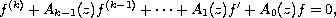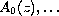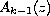Electron. J. Diff. Eqns., Vol. 2009(2009), No. 70, pp. 1-10.

### Growth and oscillation of solutions to linear differential equations with entire coefficients having the same order Benharrat Belaidi

Abstract:
In this article, we investigate the growth and fixed points of solutions of the differential equationwhere,are entire functions. Some estimates are given for the iterated order and iterated exponent of convergence of fixed points of solutions of the above equation when most of the coefficients have the same order with each other.

Submitted February 17, 2009. Published June 1, 2009.
Math Subject Classifications: 34M10, 30D35.
Key Words: Linear differential equation; growth of entire function; iterated order.

Show me the PDF file (248 KB), TEX file, and other files for this article.Benharrat Belaïdi Department of Mathematics Laboratory of Pure and Applied Mathematics University of Mostaganem, B. P. 227 Mostaganem, Algeria email: belaidi@univ-mosta.dz, belaidibenharrat@yahoo.fr